# FRETBursts - ns-ALEX example¶

This notebook is part of a tutorial series for the FRETBursts burst analysis software.

For a step-by-step introduction to FRETBursts usage please refer to us-ALEX smFRET burst analysis.

In this notebook we present a typical FRETBursts workflow for ns-ALEX smFRET burst analysis.

While FRETBursts does not specifically includes functions for fitting TCSPC fluorescence decays, a fitting with exponential decays and IRF deconvolution can be easily performed using standard python libraries. For an example and a brief discussion see the notebook Lifetime decay fit.

In :
from fretbursts import *

 - Optimized (cython) burst search loaded.
- Optimized (cython) photon counting loaded.
--------------------------------------------------------------
You are running FRETBursts (version 0.6.4).

If you use this software please cite the following paper:

FRETBursts: An Open Source Toolkit for Analysis of Freely-Diffusing Single-Molecule FRET
Ingargiola et al. (2016). http://dx.doi.org/10.1371/journal.pone.0160716

--------------------------------------------------------------

In :
sns = init_notebook()


The full list of smFRET measurements used in the FRETBursts tutorials can be found on Figshare.

Here we download the ns-ALEX data-file and put it in a folder named data, inside the notebook folder. For this purpose we use the download_file function provided by FRETBursts:

In :
url = 'http://files.figshare.com/2182602/dsdna_d7_d17_50_50_1.hdf5'

In :
download_file(url, save_dir='./data')

URL:  http://files.figshare.com/2182602/dsdna_d7_d17_50_50_1.hdf5
File: dsdna_d7_d17_50_50_1.hdf5


In :
filename = './data/dsdna_d7_d17_50_50_1.hdf5'
filename

Out:
'./data/dsdna_d7_d17_50_50_1.hdf5'

## Selecting a data file¶

Alternatively you can use an open-file dialog in order to select a data file:

In :
# filename = OpenFileDialog()
# filename

In :
import os
if os.path.isfile(filename):
print("Perfect, I found the file!")
else:
print("Sorry, I can't find the file:\n%s" % filename)

Perfect, I found the file!


Here we load the file and we set the alternation parameters:

In :
d = loader.photon_hdf5(filename)

In :
d.time_max

Out:
2268.2743759426116
In :
d.det_t

Out:
[array([1, 6, 6, ..., 6, 6, 6], dtype=uint8)]
In :
print("Detector    Counts")
print("--------   --------")
for det, count in zip(*np.unique(d.det_t, return_counts=True)):
print("%8d   %8d" % (det, count))

Detector    Counts
--------   --------
0          1
1          1
4    3345380
6    6371466

In :
#d.add(A_ON=(200, 1500), D_ON=(1750, 3200), det_donor_accept=(4, 6))

In :
d.nanotimes_t

Out:
[array([3327,  372,  579, ..., 2553, 1182,  316], dtype=uint16)]
In :
bpl.plot_alternation_hist(d)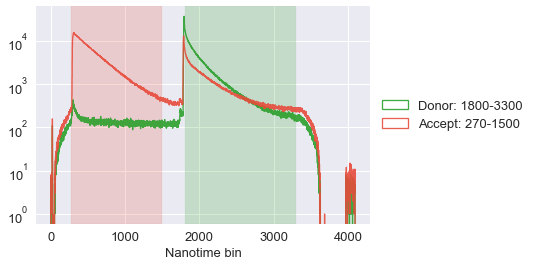Execute the previous 2 cells until you get a satisfying selection for the excitation periods. Then run the following to apply the parameters:

In :
loader.alex_apply_period(d)

# Total photons (after ALEX selection):     8,862,749
#  D  photons in D+A excitation periods:    2,866,446
#  A  photons in D+A excitation periods:    5,996,303
# D+A photons in  D  excitation period:     4,093,146
# D+A photons in  A  excitation period:     4,769,603



## Burst search and selection¶

In :
d.calc_bg(fun=bg.exp_fit, time_s=30, tail_min_us='auto', F_bg=1.7)

 - Calculating BG rates ... [DONE]

In :
dplot(d, timetrace_bg)

Out:
<matplotlib.axes._subplots.AxesSubplot at 0x124652240>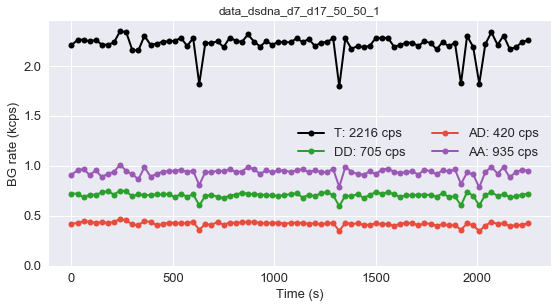In :
dplot(d, timetrace)
xlim(1, 2)
ylim(-50, 50)

Out:
(-50, 50)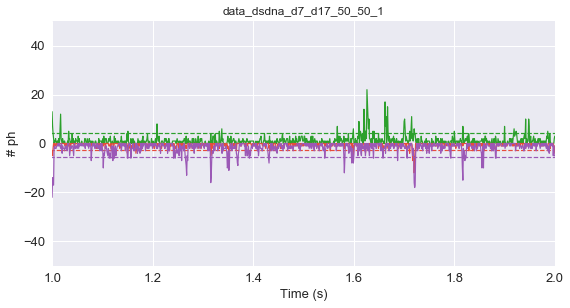In :
d.burst_search()

 - Performing burst search (verbose=False) ...[DONE]
- Calculating burst periods ...[DONE]
- Counting D and A ph and calculating FRET ...
- Applying background correction.
[DONE Counting D/A]

In :
ds = d.select_bursts(select_bursts.size, th1=30)

In :
alex_jointplot(ds)

Out:
<seaborn.axisgrid.JointGrid at 0x12418f828>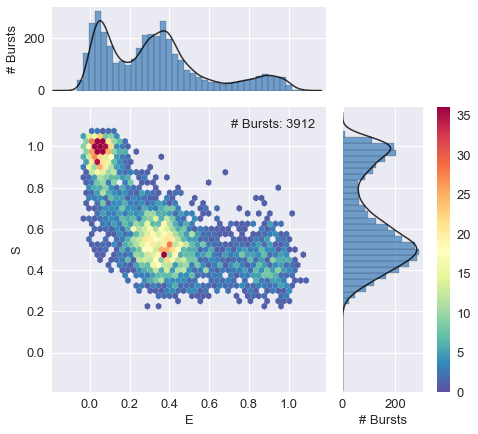In :
ds.leakage = 0.05

   - Applying background correction.
- Applying leakage correction.

In :
alex_jointplot(ds)

Out:
<seaborn.axisgrid.JointGrid at 0x124191c18>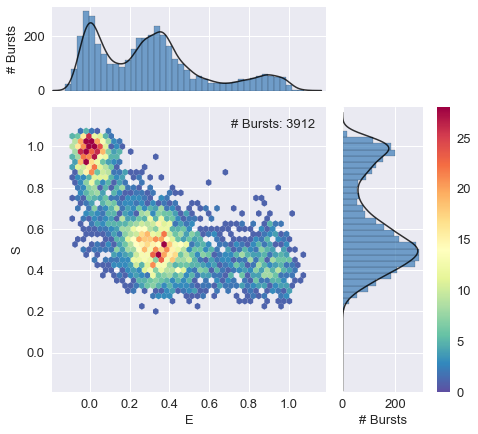In :
dplot(ds, hist_fret, show_kde=True)

Out:
<matplotlib.axes._subplots.AxesSubplot at 0x11db15cc0>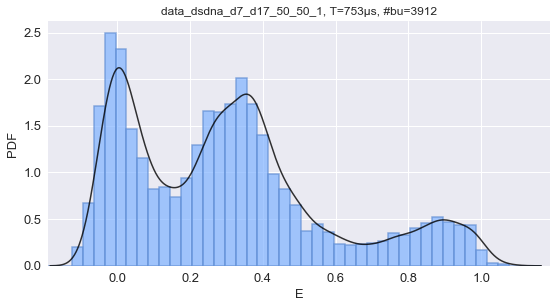## Nanotimes¶

The nanotimes for the measurement is saved in the .nanotimes attribute of the Data() object (here either d or ds).

As an example here we get the array of nanotimes for all photons, donor emission and acceptor emission:

In :
d.nanotimes

Out:
[array([ 372,  579, 1926, ..., 2553, 1182,  316], dtype=uint16)]
In :
nanotimes = d.nanotimes
nanotimes_d = nanotimes[d.get_D_em()]
nanotimes_a = nanotimes[d.get_A_em()]


We can plot the histogram for this 3 nanotimes:

In :
hist_params = dict(bins=range(4096), histtype='step', alpha=0.6, lw=1.5)
hist(nanotimes, color='k', label='Total ph.', **hist_params)
hist(nanotimes_d, color='g', label='D. em. ph.', **hist_params)
hist(nanotimes_a, color='r', label='A. em. ph.', **hist_params)
plt.legend()
plt.yscale('log')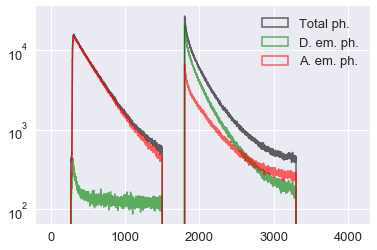We can also select only nanotimes of photons inside bursts. Here, as an example, we will use the ds variable that contains a selection of bursts.

First we compute a selection mask (a boolean array) for photons inside bursts:

In :
ph_in_bursts_mask = d.ph_in_bursts_mask_ich()


Then we apply this selection to the nanotimes array. To get the donor- and acceptor-emission nanotimes we combine the in-bursts selection mask (ph_in_bursts_mask) with the donor or acceptor emission mask (that we get with .get_D_em() and .get_D_em()):

In :
bursts_nanotimes_t = nanotimes[ph_in_bursts_mask]


And, as before, we can histogram the nanotimes:

In :
hist_params = dict(bins=range(4096), histtype='step', alpha=0.6, lw=1.5)
hist(bursts_nanotimes_t, color='k', label='Total ph.', **hist_params)
hist(bursts_nanotimes_d, color='g', label='D. em. ph.', **hist_params)
hist(bursts_nanotimes_a, color='r', label='A. em. ph.', **hist_params)
plt.legend()
plt.yscale('log')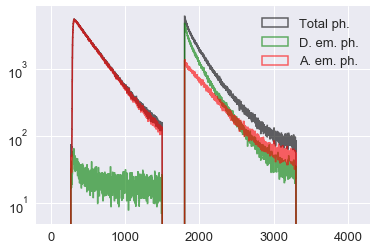## Saving to a file¶

Saving some of all these arrays to file is straightforward.

### Save array to txt comma-separed-values¶

To save a single array to a file we can use the .tofile method:

In :
nanotimes.tofile('nanotimes_t.csv', sep=',\n')  # save in CSV txt format


### Save to legacy MATLAB format¶

To save a set of arrays in MATLAB format we can use the scipy.io.savemat function.

Here we save 3 arrays bursts_nanotimes_t, bursts_nanotimes_d and bursts_nanotimes_a to a file called bursts_nanotimes.mat:

In :
from scipy.io import savemat

In :
savemat('bursts_nanotimes.mat',
dict(bn_t=bursts_nanotimes_t,
bn_d=bursts_nanotimes_d,
bn_a=bursts_nanotimes_a,))


When loaded in MATLAB the arrays will be named bn_t, bn_d and bn_a.

In :


In :


In :


In :



Executed: Tue Jul 11 21:53:19 2017

Duration: 22 seconds.

Autogenerated from: FRETBursts - ns-ALEX example.ipynb